Get instant live expert help with Excel or Google Sheets“My Excelchat expert helped me in less than 20 minutes, saving me what would have been 5 hours of work!”

#### Post your problem and you'll get expert help in seconds

Your message must be at least 40 characters
Our professional experts are available now. Your privacy is guaranteed.

# Excel MROUND Function

We use the MROUND function to round off a given set of numbers either up or down to the nearest specified multiple. The steps below will walk through the process.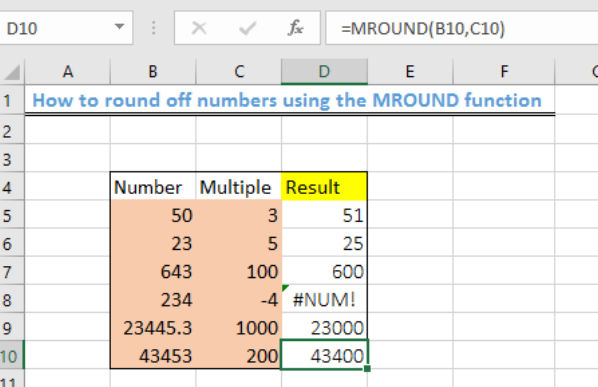Figure 1- Result of rounding off numbers using the MROUND function

## General formula

`=MROUND(num,multiple)`

## Formula

`=MROUND (B5,C5)`

## Setting up the Data

The objective is to round off each number using the multiples as shown in figure 2

• We will input the numbers in the range B5:B10 and titled them as “Number.
• We will put the multiples in C5:C10 and title them as “Multiple.
• Column D will contain the results after applying the MROUND function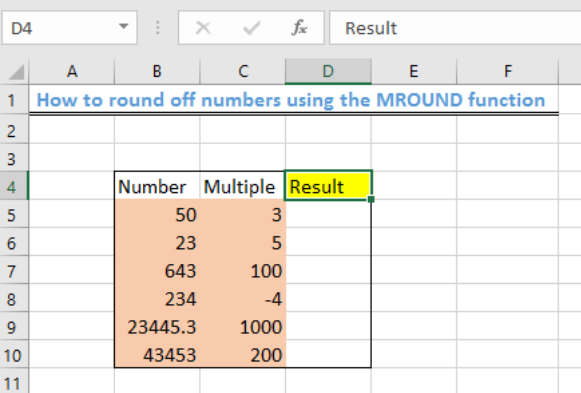Figure 2- Setting up the Data

## Applying the MROUND function

• We will click on Cell D5

• We will insert the formula: =MROUND (B5,C5) into Cell D5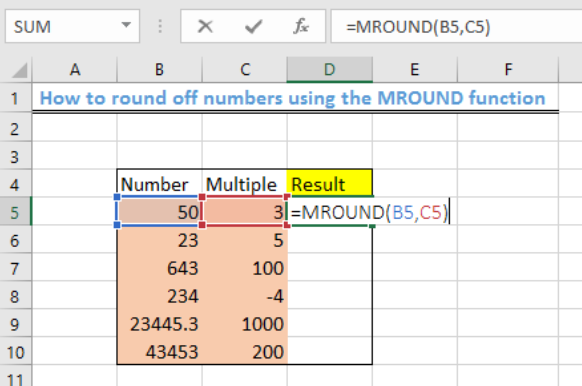Figure 3 – Applying the MROUND function

• We will press the Enter key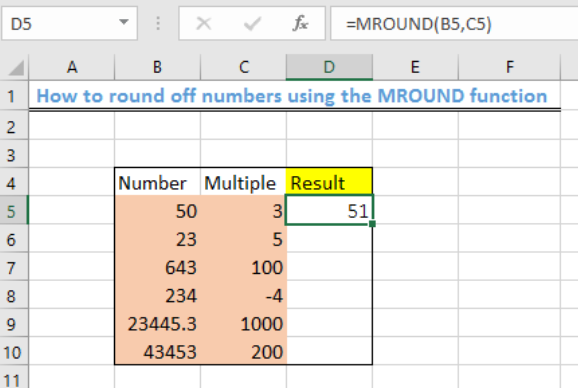Figure 4- How to round off numbers using MROUND function

• Using the fill handle, we will copy the formula to the other cells of the Result column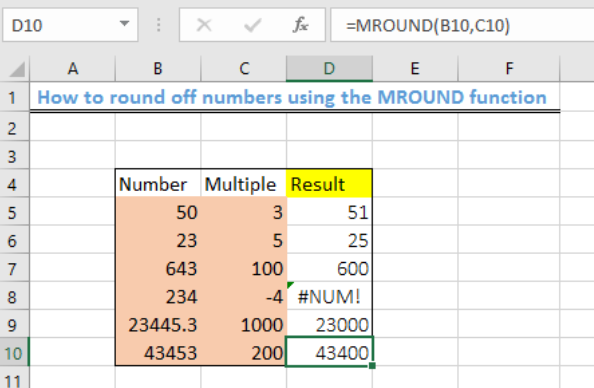Figure 5 – Result of rounding off numbers using the MROUND function

## Explanation

`=MROUND (num,multiple)`

The MROUND function rounds off numbers (“num”) to the nearest multiple that is specified. The MROUND function always rounds away from zero and the rounding will occur if the remainder from the left when dividing the number by the multiple is greater than or equal to half of the multiple values. Multiple must also have the same sign as the number. If the numbers and multiples do not have the same sign, then the #NUM error will be displayed as shown in figure 4, Cell D8.

## Instant Connection to an Expert through our Excelchat Service

Most of the time, the problem you will need to solve will be more complex than a simple application of a formula or function. If you want to save hours of research and frustration, try our live Excelchat service! Our Excel Experts are available 24/7 to answer any Excel question you may have. We guarantee a connection within 30 seconds and a customized solution within 20 minutes.

### Did this post not answer your question? Get a solution from connecting with the expert.Another blog reader asked this question today on Excelchat:## Subscribe to Excelchat.coAnother blog reader asked this question today on Excelchat: